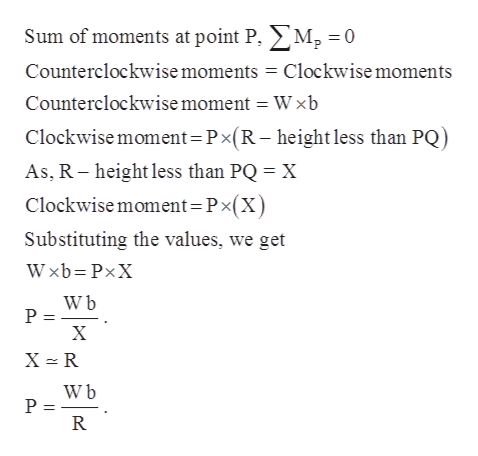# RWP-2bFig. P4.170

Question

The homogenous cylinder of weight W and radius rests  in a groove of width 2b. Determine the smallest force P required to roll the cylinder out of the groove. Assume that there is enough friction to prevent slipping.

check_circleExpert Solution
Step 1

The following information has been provided

The homogeneous cylinder of weight W and radius R rests in a groove

of width 2b. Assume that there is enough friction to prevent slipping.

To find: The smallest force P required to roll the cylinder out of

the groove.

Step 2

The following diagram will explain the essential force system required

Step 3

For calculating the minimum force that will roll out the wheel from the groove can be calculated by finding out...help_outlineImage TranscriptioncloseSum of moments at point P. M2 = 0 Counterclockwise moments Clockwisemoments Counterclockwise moment = Wxb Clockwise moment Px(R- height less than PQ) As, R height less than PQ X Clockwise moment=Px(X) Substituting the values, we get Wxb PxX W b P = X X R W b P = R fullscreen

### Want to see the full answer?

See Solution

#### Want to see this answer and more?

Solutions are written by subject experts who are available 24/7. Questions are typically answered within 1 hour*

See Solution
*Response times may vary by subject and question
Tagged in

### Civil Engineering